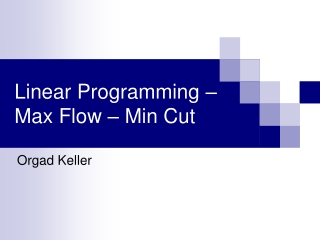DownloadDownload PresentationLinear Programming – Max Flow – Min Cut

# Linear Programming – Max Flow – Min Cut

Download Presentation## Linear Programming – Max Flow – Min Cut

- - - - - - - - - - - - - - - - - - - - - - - - - - - E N D - - - - - - - - - - - - - - - - - - - - - - - - - - -
##### Presentation Transcript

1. Linear Programming – Max Flow – Min Cut Orgad Keller

2. Linear Programming • We have an objective function: • Subject to constraints: Orgad Keller - Algorithms 2

3. Linear Programming • Given all parameters • we want to find the optimal Orgad Keller - Algorithms 2 -

4. Linear Programming • It is easier to present the problem with a matrix and vectors: Orgad Keller - Algorithms 2 -

5. The Dual Problem • Given the Primal Problem: • It’s Dual Problem is defined as: Orgad Keller - Algorithms 2 -

6. Strong Duality Theorem • Given a problem and it’s dual problem, then: • In other words, the optimal objective function’s value for the primal problem, is equal to the optimal objective function’s value for the dual problem. Orgad Keller - Algorithms 2 -

7. Maximum Flow • As you remember from Algorithms I • Given a directed graph , two vertices and a capacity for each edge • We want to find a flow function so that the flow value is maximal Orgad Keller - Algorithms 2 -

8. Maximum Flow • But, we are subject to some rules: • What goes in must come out: • Capacity restrictions: Orgad Keller - Algorithms 2 -

9. Max Flow with Linear Programming • We’ll show by a simple example: 1 3 1 3 2 Orgad Keller - Algorithms 2 -

10. Max Flow with Linear Programming • We want to present the example in the form: • Subject to: Orgad Keller - Algorithms 2 -

11. 3 1 1 3 2 But we don’t permit equalities Max Flow with Linear Programming • Formally: Orgad Keller - Algorithms 2 -

12. Max Flow with Linear Programming 3 1 1 • So we’ll add another edge, and change the problem’s representation a little. 3 2 Orgad Keller - Algorithms 2 -

13. Orgad Keller - Algorithms 2 -

14. Orgad Keller - Algorithms 2 -

15. Minimum Cut • As you remember from Algorithms I • Given a directed graph , two vertices and a weight for each edge • We want to find a minimal-weight subset of edges such that if we’ll remove them, we won’t be able to travel from to . Orgad Keller - Algorithms 2 -

16. Minimum Cut • In other words: • We’ll choose , where: , , such that the cut value, is minimal. Orgad Keller - Algorithms 2 -

17. Min Cut with Linear Programming • Going back to the example: 1 3 1 3 2 Orgad Keller - Algorithms 2 -

18. 3 1 1 3 2 This look like And we know that Min Cut with Linear Programming • What about: • Is that enough? • We haven’t ensured paths from to are cut. Orgad Keller - Algorithms 2 -

19. 3 1 1 3 2 Min Cut with Linear Programming • Beside a variable for every edge , we’ll want a variable for every vertex , such that: Orgad Keller - Algorithms 2 -

20. 3 1 1 3 2 Min Cut with Linear Programming • Let’s take for instance: • If is in the cut , that means that • If is not in the cut , that means that either or or • So it is the same to constrain: Orgad Keller - Algorithms 2 -

21. 3 1 1 3 2 In order to ensure Max Flow with Linear Programming • Formally: Orgad Keller - Algorithms 2 -

22. Orgad Keller - Algorithms 2 -

23. Orgad Keller - Algorithms 2 -

24. Orgad Keller - Algorithms 2 -

25. Max Flow – Min Cut Theorem • We now see that the problems are dual • So also according to the Strong Duality Theorem, Max Flow = Min Cut Orgad Keller - Algorithms 2 -

26. Integer Values • We want integer values for the variables, but this is LP, not IP! • So how do we know that the solution will yield integer values for the variables? • Theroem: If the constraints matrix is totally unimodular and the right hand side is comprised of integers, then it’s easy to find an integer solution. Orgad Keller - Algorithms 2 -

27. Unimodular / Totally Unimodular • Definition: A Unimodular matrix is a square matrix whose determinant is 0, 1 or -1. • Definition: A Totally Unimodular matrix is a matrix whose every non-singular square submatrix is unimodular. Orgad Keller - Algorithms 2 -